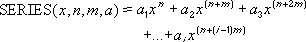## Excel Office

Excel How Tos, Tutorials, Tips & Tricks, Shortcuts

# SERIESSUM function: Description, Usage, Syntax, Examples and Explanation

## What is SERIESSUM function in Excel?

SERIESSUM function is one of the Math and Trig functions in Microsoft Excel that returns the sum of a power series based on the formula:Many functions can be approximated by a power series expansion.

## Syntax of SERIESSUM function

SERIESSUM(x, n, m, coefficients)

The SERIESSUM function syntax has the following arguments:

• X: The input value to the power series.
• N: The initial power to which you want to raise x.
• M: The step by which to increase n for each term in the series.
• Coefficients: A set of coefficients by which each successive power of x is multiplied. The number of values in coefficients determines the number of terms in the power series. For example, if there are three values in coefficients, then there will be three terms in the power series.

## SERIESSUM formula explanation

If any argument is nonnumeric, SERIESSUM returns the #VALUE! error value.

## Example of SERIESSUM function

Steps to follow:

1. Open a new Excel worksheet.

2. Copy data in the following table below and paste it in cell A1

Note: For formulas to show results, select them, press F2 key on your keyboard and then press Enter.

You can adjust the column widths to see all the data, if need be.

 Data Coefficients as numbers Coefficients as formulae 0.785398163 =PI()/4 1 1 -0.5 =-1/FACT(2) 0.041666667 =1/FACT(4) -0.001388889 =-1/FACT(6) Formula Description (Result) Result =SERIESSUM(A3,0,2,A4:A7) Approximation to the cosine of Pi/4 radians, or 45 degrees (0.707103) 0.707103